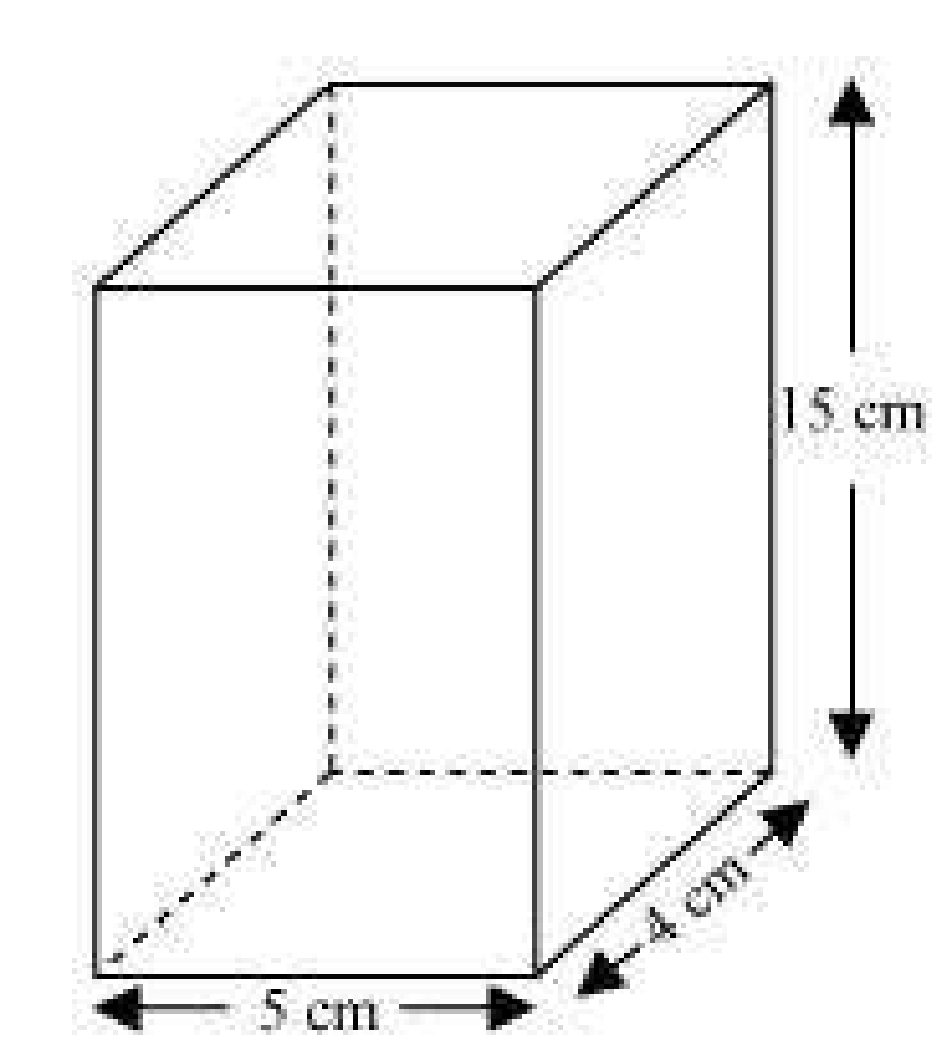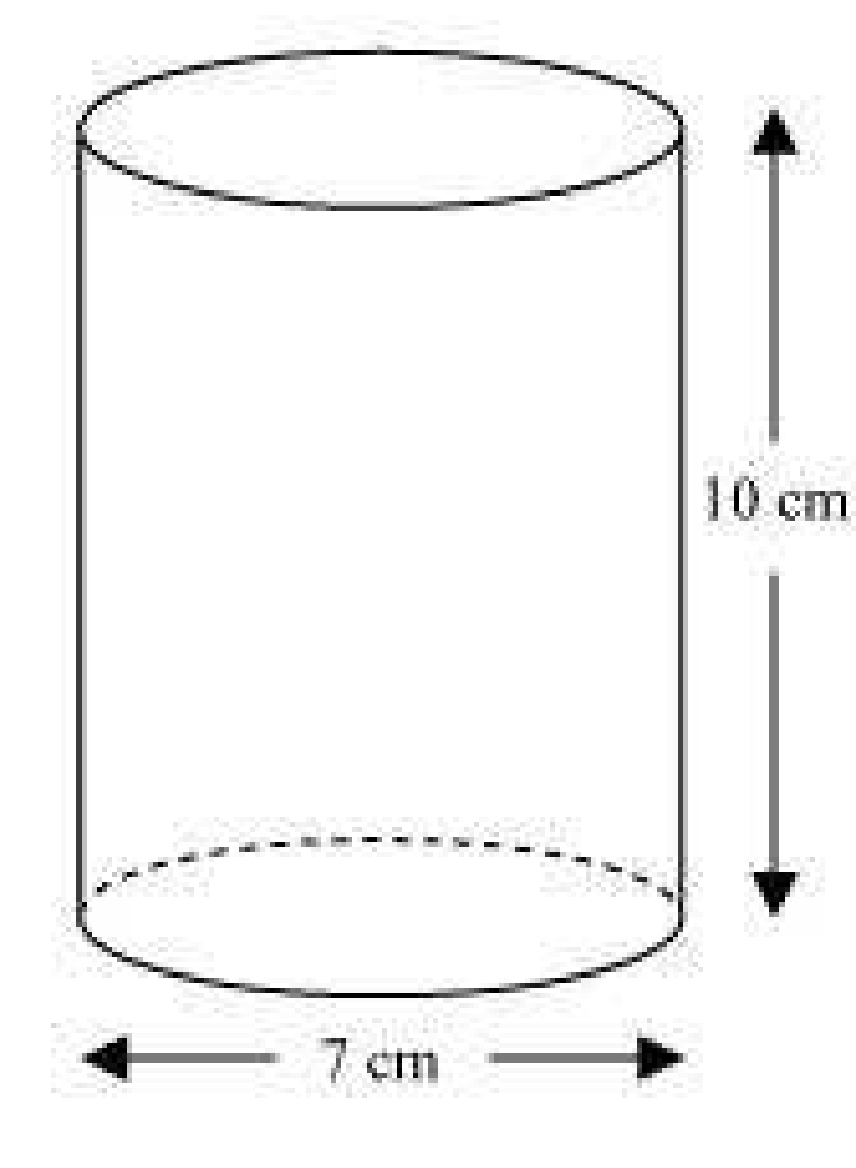Guru

# A soft drink is available in two packs – (i) a tin can with a rectangular base of length 5cm and width 4cm, having a height of 15 cm and (ii) a plastic cylinder with circular base of diameter 7cm and height 10cm. Which container has greater capacity and by how much? (Assume π=22/7) Q.3

• 0

How can i solve this tough question of class 9th ncert of Surface areas and Volumes of math of exercise 13.6   of question no.3 . Give me the best and simple way for solving this question. A soft drink is available in two packs – (i) a tin can with a rectangular base of length 5cm and width 4cm, having a height of 15 cm and (ii) a plastic cylinder with circular base of diameter 7cm and height 10cm. Which container has greater capacity and by how much? (Assume π=22/7)

Share

1. tin can will be cuboidal in shapeDimensions of tin can are

Length, l = 5 cm

Breadth, b = 4 cm

Height, h = 15 cm

Capacity of tin can = l×b×h= (5×4×15) cm3 = 300 cm3

1. Plastic cylinder will be cylindrical in shape.Dimensions of plastic can are:

Radius of circular end of plastic cylinder, r = 3.5cm

Height , H = 10 cm

Capacity of plastic cylinder = πr2H

Capacity of plastic cylinder = (22/7)×(3.5)2×10 = 385

Capacity of plastic cylinder is 385 cm3

From results of (i) and (ii), plastic cylinder has more capacity.

Difference in capacity = (385-300) cm3 = 85cm3

• 0